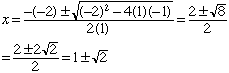Quandaries and Queries Subject: Quadratic Equations Name: Katie Who is asking: Student Level: All Hi, I am having problems with my homework. As I have this one math problem that is just bugging the hell outta me and I cannot figure it out for the life of me. 1)x-2x-1 =0 Find both roots. You must simplify both of your roots. This equation requires the use of the Quadratic Formula. I have tried everything for this and I am still either getting the wrong answers, and end up getting really frustrated. If you could find the answers and tell me how you did it I would greatly appreciate it! Then the second problem is 2) The square of a certain negative number is equal to 70 decreased by 3 times the number. Find the answer! On this one I set it up but still came out with the wrong answer. So if you could help me on this one I would greatly appreciate it! Sincerely, Katie Hi Katie, Is the first problem x2-2x-1=0? If so then by the quadratic formula I getFor the second problem if the number is x then x2 = 70 - 3x and hence x2 + 3x - 70 = 0 Which factors as (x - 7)(x + 10) = 0 Thus x = 7 or x = -10. Since you are looking for a negative number, x = -10. Penny Go to Math Central